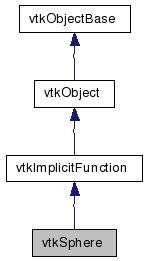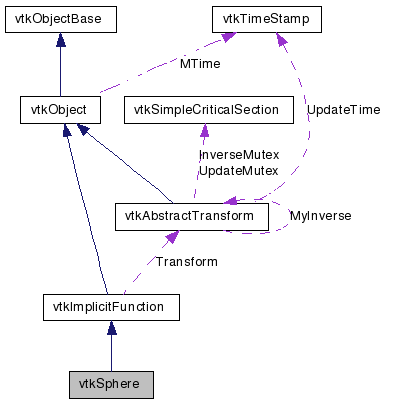# vtkSphere Class Reference

`#include <vtkSphere.h>`

Inheritance diagram for vtkSphere:[legend]
Collaboration diagram for vtkSphere:[legend]

## Detailed Description

implicit function for a sphere

vtkSphere computes the implicit function and/or gradient for a sphere. vtkSphere is a concrete implementation of vtkImplicitFunction. Additional methods are available for sphere-related computations, such as computing bounding spheres for a set of points, or set of spheres.

Tests:
vtkSphere (Tests)

Definition at line 32 of file vtkSphere.h.

## Public Types

typedef vtkImplicitFunction Superclass

## Public Member Functions

virtual const char * GetClassName ()
virtual int IsA (const char *type)
void PrintSelf (ostream &os, vtkIndent indent)
void EvaluateGradient (double x, double n)
double EvaluateFunction (double x)
double EvaluateFunction (double x, double y, double z)
virtual void SetCenter (double, double, double)
virtual void SetCenter (double)
virtual double * GetCenter ()
virtual void GetCenter (double data)

## Static Public Member Functions

static int IsTypeOf (const char *type)
static vtkSphereSafeDownCast (vtkObject *o)
static vtkSphereNew ()
static void ComputeBoundingSphere (float *pts, vtkIdType numPts, float sphere, vtkIdType hints)
static void ComputeBoundingSphere (double *pts, vtkIdType numPts, double sphere, vtkIdType hints)
static void ComputeBoundingSphere (float **spheres, vtkIdType numSpheres, float sphere, vtkIdType hints)
static void ComputeBoundingSphere (double **spheres, vtkIdType numSpheres, double sphere, vtkIdType hints)

vtkSphere ()
~vtkSphere ()

## Protected Attributes

double Center 

## Member Typedef Documentation

Reimplemented from vtkImplicitFunction.

Definition at line 35 of file vtkSphere.h.

## Constructor & Destructor Documentation

 vtkSphere::vtkSphere ( ) ` [protected]`

 vtkSphere::~vtkSphere ( ) ` [inline, protected]`

Definition at line 91 of file vtkSphere.h.

## Member Function Documentation

 virtual const char* vtkSphere::GetClassName ( ) ` [virtual]`

Reimplemented from vtkImplicitFunction.

 static int vtkSphere::IsTypeOf ( const char * name ) ` [static]`

Return 1 if this class type is the same type of (or a subclass of) the named class. Returns 0 otherwise. This method works in combination with vtkTypeRevisionMacro found in vtkSetGet.h.

Reimplemented from vtkImplicitFunction.

 virtual int vtkSphere::IsA ( const char * name ) ` [virtual]`

Return 1 if this class is the same type of (or a subclass of) the named class. Returns 0 otherwise. This method works in combination with vtkTypeRevisionMacro found in vtkSetGet.h.

Reimplemented from vtkImplicitFunction.

 static vtkSphere* vtkSphere::SafeDownCast ( vtkObject * o ) ` [static]`

Reimplemented from vtkImplicitFunction.

 void vtkSphere::PrintSelf ( ostream & os, vtkIndent indent ) ` [virtual]`

Methods invoked by print to print information about the object including superclasses. Typically not called by the user (use Print() instead) but used in the hierarchical print process to combine the output of several classes.

Reimplemented from vtkImplicitFunction.

 static vtkSphere* vtkSphere::New ( ) ` [static]`

Construct sphere with center at (0,0,0) and radius=0.5.

Reimplemented from vtkObject.

 double vtkSphere::EvaluateFunction ( double x ) ` [virtual]`

Evaluate sphere equation ((x-x0)^2 + (y-y0)^2 + (z-z0)^2) - R^2.

Implements vtkImplicitFunction.

 double vtkSphere::EvaluateFunction ( double x, double y, double z ) ` [inline]`

Evaluate sphere equation ((x-x0)^2 + (y-y0)^2 + (z-z0)^2) - R^2.

Reimplemented from vtkImplicitFunction.

Definition at line 44 of file vtkSphere.h.

 void vtkSphere::EvaluateGradient ( double x, double n ) ` [virtual]`

Implements vtkImplicitFunction.

 virtual void vtkSphere::SetRadius ( double ) ` [virtual]`

Set / get the radius of the sphere.

 virtual double vtkSphere::GetRadius ( ) ` [virtual]`

Set / get the radius of the sphere.

 virtual void vtkSphere::SetCenter ( double , double , double ) ` [virtual]`

Set / get the center of the sphere.

 virtual void vtkSphere::SetCenter ( double  ) ` [virtual]`

Set / get the center of the sphere.

 virtual double* vtkSphere::GetCenter ( ) ` [virtual]`

Set / get the center of the sphere.

 virtual void vtkSphere::GetCenter ( double data ) ` [virtual]`

Set / get the center of the sphere.

 static void vtkSphere::ComputeBoundingSphere ( float * pts, vtkIdType numPts, float sphere, vtkIdType hints ) ` [static]`

Create a bounding sphere from a set of points. The set of points is defined by an array of doubles, in the order of x-y-z (which repeats for each point). An optional hints array provides a guess for the initial bounding sphere; the two values in the hints array are the two points expected to be the furthest apart. The output sphere consists of a center (x-y-z) and a radius.

 static void vtkSphere::ComputeBoundingSphere ( double * pts, vtkIdType numPts, double sphere, vtkIdType hints ) ` [static]`

Create a bounding sphere from a set of points. The set of points is defined by an array of doubles, in the order of x-y-z (which repeats for each point). An optional hints array provides a guess for the initial bounding sphere; the two values in the hints array are the two points expected to be the furthest apart. The output sphere consists of a center (x-y-z) and a radius.

 static void vtkSphere::ComputeBoundingSphere ( float ** spheres, vtkIdType numSpheres, float sphere, vtkIdType hints ) ` [static]`

Create a bounding sphere from a set of spheres. The set of input spheres is defined by an array of pointers to spheres. Each sphere is defined by the 4-tuple: center(x-y-z)+radius. An optional hints array provides a guess for the initial bounding sphere; the two values in the hints array are the two spheres expected to be the furthest apart. The output sphere consists of a center (x-y-z) and a radius.

 static void vtkSphere::ComputeBoundingSphere ( double ** spheres, vtkIdType numSpheres, double sphere, vtkIdType hints ) ` [static]`

Create a bounding sphere from a set of spheres. The set of input spheres is defined by an array of pointers to spheres. Each sphere is defined by the 4-tuple: center(x-y-z)+radius. An optional hints array provides a guess for the initial bounding sphere; the two values in the hints array are the two spheres expected to be the furthest apart. The output sphere consists of a center (x-y-z) and a radius.

## Member Data Documentation

 double vtkSphere::Radius` [protected]`

Definition at line 91 of file vtkSphere.h.

 double vtkSphere::Center` [protected]`

Definition at line 94 of file vtkSphere.h.

The documentation for this class was generated from the following file:

Generated on Wed Jun 3 19:35:13 2009 for VTK by1.5.6